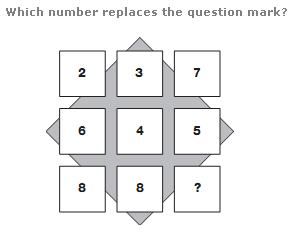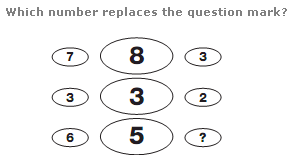# Puzzles - Number puzzles

Exercise : Number puzzles
157.:
2
Explanation
:
Reading each line of the diagram as a 3 digit number, add the top and middle lines together to give the result on the bottom line.

158.:
1
Explanation
:
In each row, add the left and right hand numbers together and subtract 2 to give the number in the centre of the row.

159.